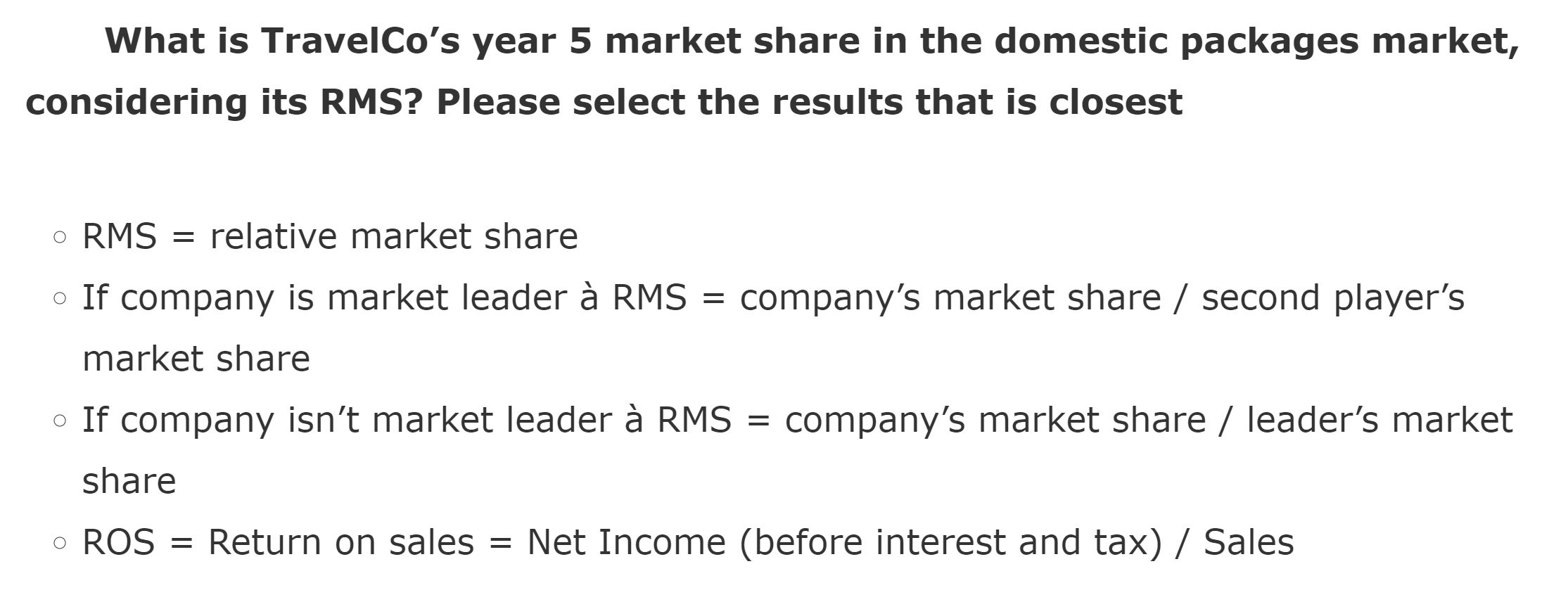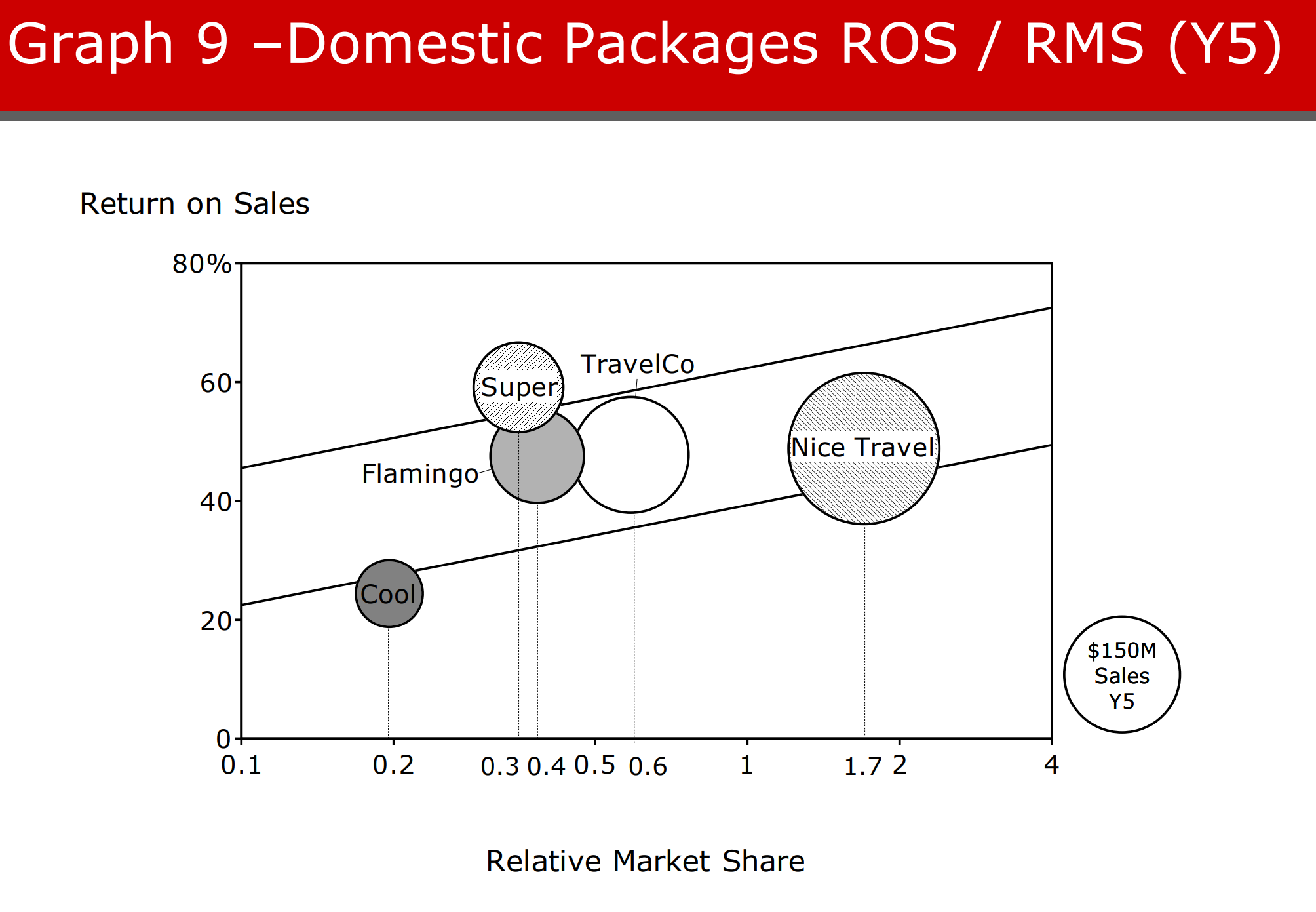# Bain Question - Business Case Sample

Bain Bain & Company business case Case math test preparation
Recent activity on Aug 16, 2018
5.9 k Views

Hi!

I would appreciate if someone could help me with this question. (it's from a Bain sample case)

I've attached the chart (which has all the info needed) and the question.

The answer is 20% but I can not come up with that number! if anyone could give me a hint..

Thanks!!!(edited)

• Date ascending
• Date descending

0.6/(0.2+0.3+0.4+0.5+0.6+1)

Is it a rounded number? Why? I got 24% by calculating the full formulas given in intro.

use The formula above, the answer will be 0.2

(edited)

Hello! Could someone please give me a hint on how to get to this answer? Thank you

Here's my guess and I'm not sure if this actually makes sense. RMS = MS_company / MS_nice_travel for each company. Then RMS * MS_nice_travel = MS_company for each company. We can take MS_travel_co divided by the sum of RMS * MS_nice_travel for each company. You can organize like this:

MS_travel_co = (0.6 * MS_nice_travel) ÷ ((0.2 + 0.3 + 0.4 + 0.6 + 1) * MS_nice_travel)

Yeah I think there’s something wrong with it

The step by step approach is by referring to the ratios given.

Travelco/Nice = 0.6

Nice/Travelco = 1.7

Super/Nice = 0.3

Flamingo/Nice = 0.4

Cool/Nice = 0.2

Then, from the x axis, we know Nice + Super + Travelco + Flamingo + Cool = 150.

Just by doing math manipulations, we can obtain Nice = 60; then Travelco is 0.6 X Nice, which is 36. Finally, Travelco market share = 36/150 = 24%.

Btw, may I know where I can get the this full case? I looks interesting. :)

Express every individual company market share as a function of NiceTravel’s market share (using RMS), then setup an equation to solve for Nice Travels market share (40%). From there onwards, use the expression for Travel Co market share relative to Nice Travel to solve for Travel Co market share.

M.tc=0.6M.nt

Thus M.tc=0.6x40% = 24%